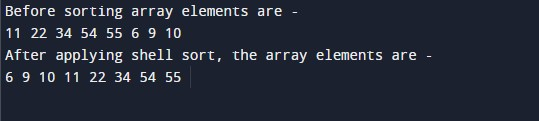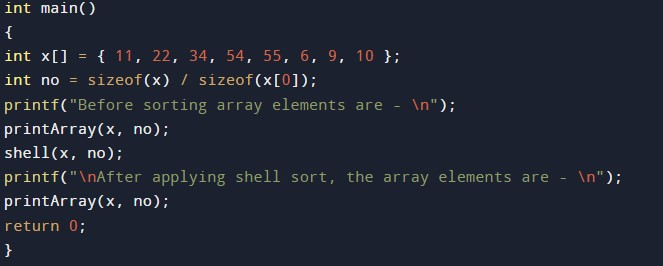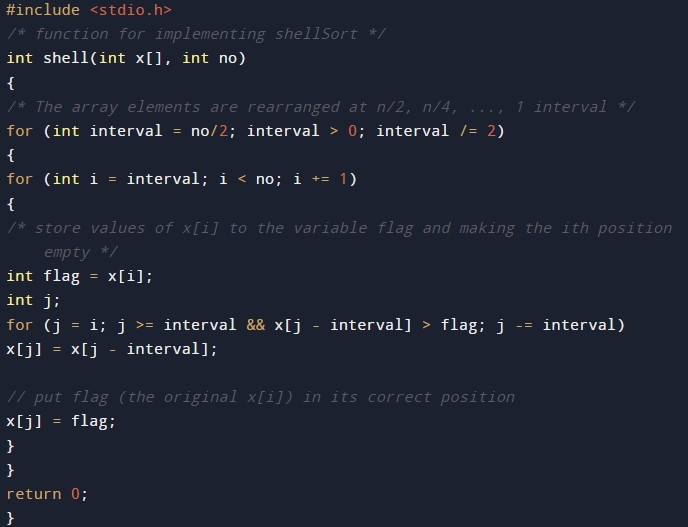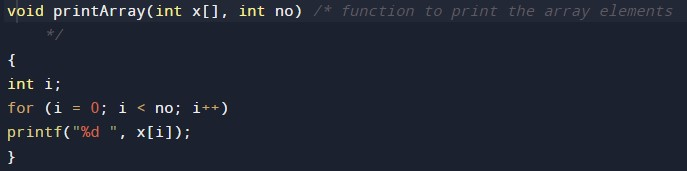# C Program to implement Shell sort Algorithm

In this tutorial you will learn about the C Program to implement Shell sort Algorithm and its application with practical example.

## C Program to implement Shell sort Algorithm

In this tutorial, we will learn to create a C program that will do Shell sort using C programming.

## Prerequisites

Before starting this tutorial we assume that you are best aware of the following C programming topics:

• Operators in C Programming.
• Basic Input and Output function in C Programming.
• Basic C programming.
• For loop in C Programming.
• Creating and Using the user-defined function in C programming.

## What is Shell Sort?

In every programming language, the sorting of data is a very important factor. Sorting works are done mainly with the techniques available in C Language. Many different techniques for sorting are available to work with but in this tutorial, we will focus on the Shell Sort Technique.

The Shell Sort is an evolved version of the insertion sort. Well will pick the data from an index point of array is and swap it with a faraway position index. Then data interchange between 2 locations from the array and doesn’t need to be too close to be swapped.

## Output:-In the above program, we have first declared and initialized a set of variables required in the program.

• no = it will hold the size of an array.
• x[]= it will hold the elements in an array.
• i= it will hold the integer value to the control array.
• j= it will hold the integer value to the control array.

After this, we make the main function for our program and define variables calling functions.The Shell Sorting function bodyThe printing array function bodyIn this tutorial we have learn about the C Program to implement Shell sort Algorithm and its application with practical example. I hope you will like this tutorial.